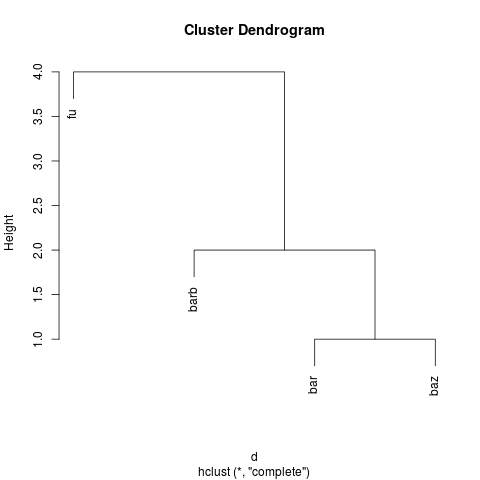[This article was first published on Mark van der Loo » R, and kindly contributed to R-bloggers]. (You can report issue about the content on this page here)
Want to share your content on R-bloggers? click here if you have a blog, or here if you don't.

On 24-06-2015 stringdist 0.9.2 was accepted on CRAN. A summary of new features can be found in the NEWS file; here I discuss the changes with some examples.

## Computing ‘dist’ objects with ‘stringdistmatrix’

The R dist object is used as input for many clustering algorithms such as cluster::hclust. It is stores the lower triangle of a matrix of distances between a vector of objects. The function stringdist::stringdistmatrix now takes a variable number of character arguments. If two vectors are given, it behaves the same as it used to.

> x <- c("fu","bar","baz","barb")
> stringdistmatrix(x,x,useNames="strings")
fu bar baz barb
fu    0   3   3    4
bar   3   0   1    1
baz   3   1   0    2
barb  4   1   2    0

However, we’re doing more work then necessary. Feeding stringdistmatrix just a single character argument yields the same information, but at half the computational and storage cost.

> stringdistmatrix(x,useNames="strings")
fu bar baz
bar   3
baz   3   1
barb  4   1   2

The output is a dist object storing only the subdiagonal triangle. This makes it particularly easy to cluster texts using any algorithm that takes a dist object as argument. Many such algorithms available in R do, for example:

d <- stringdistmatrix(x,useNames="strings")
h <- stats::hclust(d)
plot(h)(by the way, parallelizing the calculation of a lower triangle of a matrix poses an interesting exercise in index calculation. For those interested, I wrote it down)

## Better labeling of distance matrices

Distance matrices can be labeled with the input strings by setting the useNames argument in stringdistmatrix to TRUE or FALSE (the default). However, if you're computing distances between looooong strings, like complete texts it is more convenient to use the names attribute of the input vector. So, the useNames arguments now takes three different values.

> x <- c(one="fu",two="bar",three="baz",four="barb")
> y <- c(a="foo",b="fuu")
> # the default:
> stringdistmatrix(x,y,useNames="none")
[,1] [,2]
[1,]    2    1
[2,]    3    3
[3,]    3    3
[4,]    4    4
> # like useNames=TRUE
> stringdistmatrix(x,y,useNames = "strings")
foo fuu
fu     2   1
bar    3   3
baz    3   3
barb   4   4
> # use labels
> stringdistmatrix(x,y,useNames="names")
a b
one   2 1
two   3 3
three 3 3
four  4 4

## String similarities

Thanks to Jan van der Laan, a string similarity convenience function has been added. It computes the distance metric $d$ between two strings and then rescales it as $s = 1 - d/max(d)$, where the maximum possible distance $max(d)$ depends on the type of distance metric and (depending on the metric) the length of the strings.

# similarity based on the damerau-levenshtein distance
> stringsim(c("hello", "World"), c("Ola", "Mundo"),method="dl")
 0.2 0.0
# similarity based on the jaro distance
> stringsim(c("hello", "World"), c("Ola", "Mundo"),method="jw")
 0.5111111 0.4666667

Here a similarity of 0 means completely different and 1 means exactly the same (within the chosen metric).

## Deprecated arguments

The stringdistmatrix function had to option to be computed in parallel based on facilities of the parallel package. However, as of stringdist 0.9.0, all distance calculations are multicored by default.

Therefore, I'm phasing out the following options in stringdistmatrix:

• ncores (how many R-sessions should be started by parallel to compute the matrix?)
• cluster (optionally, provide your own cluster, created by parallel::makeCluster.

These argument are now ignored with a message but they'll be available untill somewhere in 2016 so users have time to adapt their code. Please mail me if you have any trouble doing so.